# Manifold graphics in SageMath¶

This notebook illustrates some plotting capabilities of manifold objects in SageMath.

More examples can be found on the SageManifolds page.

In :
%display latex


## The 2-sphere example¶

Let us consider the sphere $\mathbb{S}^2$. In SageMath, we declare it as a 2-dimensional differentiable manifold:

In :
S2 = Manifold(2, 'S^2', latex_name=r'\mathbb{S}^2', start_index=1)
print(S2)

2-dimensional differentiable manifold S^2


We then introduce the standard spherical coordinates $(\theta,\phi)$ as a chart on $\mathbb{S}^2$, denoting the chart as spher and the coordinates th and ph. We shall be lazy here and consider that the spherical chart covers the whole manifold $\mathbb{S}^2$; this amounts to authorize coordinate singularities:

In :
spher.<th,ph> = S2.chart(r'th:[0,pi]:\theta ph:[0,2*pi]:periodic:\phi')
spher

Out:
In :
spher.coord_range()

Out:

For 3D plots, we shall require the Euclidian space $\mathbb{E}^3$:

In :
E3.<X,Y,Z> = EuclideanSpace()
E3

Out:

Let us call cartesian the chart of Cartesian coordinates $(X,Y,Z)$:

In :
cartesian = E3.cartesian_coordinates()
cartesian

Out:

The canonical embedding of $\mathbb{S}^2$ into $\mathbb{E}^3$:

In :
Phi = S2.diff_map(E3, (sin(th)*cos(ph),
sin(th)*sin(ph),
cos(th)),
name='Phi', latex_name=r'\Phi')
Phi.display()

Out:

## Plot of a chart¶

Each chart is endowed with a plot() method. For instance, we may use it to plot the chart of spherical coordinates plotted in terms of itself:

In :
spher.plot(chart=spher)

Out: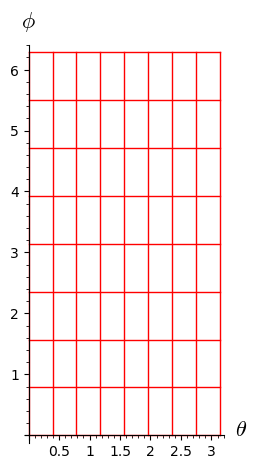More intersting is to the plot the chart of spherical coordinates in terms of $\mathbb{E}^3$'s Cartesian coordinates, via the embedding $\Phi:\; \mathbb{S}^2\to\mathbb{E}^3$:

In :
spher.plot(chart=cartesian, mapping=Phi)

Out:

For a better image, let us increase the number of coordinate values:

In :
spher.plot(chart=cartesian, mapping=Phi, number_values=11)

Out:

One can customize the color of coordinate lines:

In :
spher.plot(chart=cartesian, mapping=Phi, number_values=11,
color={th: 'red', ph: 'green'})

Out:

One can fix the value of some coordinates, thereby obtaining a partial plot:

In :
spher.plot(chart=cartesian, mapping=Phi, number_values=11,
color={th: 'red', ph: 'green'},
fixed_coords={th: pi/4})

Out:

or limit the range of some coordinates:

In :
spher.plot(chart=cartesian, mapping=Phi, number_values=11,
color={th: 'red', ph: 'green'},
ranges={ph:(0, pi)})

Out:

One can also restrict the coordinates of the ambiant chart; here is a example of plot of the chart spher in terms of the coordinates $(X,Y)$ of $\mathbb{E}^3$:

In :
spher.plot(chart=cartesian, mapping=Phi, number_values=11,
color={th: 'red', ph: 'green'},
ambient_coords=(X, Y))

Out: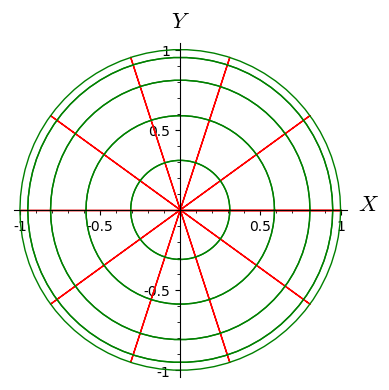Same thing with only the coordinates $(X,Z)$:

In :
spher.plot(chart=cartesian, mapping=Phi, number_values=11,
color={th: 'red', ph: 'green'},
ambient_coords=(X, Z))

Out: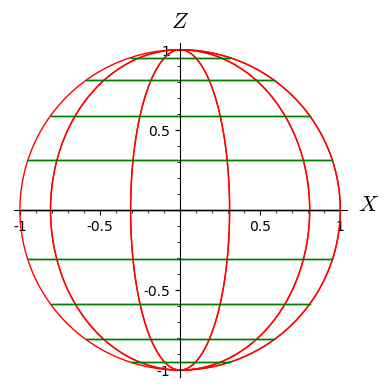Let us introduce the chart of stereographic coordinates from the North pole:

In :
stereoN.<x,y> = S2.chart()
stereoN

Out:
In :
spher_to_stereoN = spher.transition_map(stereoN,
(sin(th)*cos(ph)/(1-cos(th)),
sin(th)*sin(ph)/(1-cos(th))))
spher_to_stereoN.display()

Out:
In :
spher_to_stereoN.set_inverse(2*atan(1/sqrt(x^2+y^2)), atan2(-y,-x)+pi)

Check of the inverse coordinate transformation:
th == 2*arctan(sqrt(-cos(th) + 1)/sqrt(cos(th) + 1))  **failed**
ph == pi + arctan2(sin(ph)*sin(th)/(cos(th) - 1), cos(ph)*sin(th)/(cos(th) - 1))  **failed**
x == x  *passed*
y == y  *passed*
NB: a failed report can reflect a mere lack of simplification.


Plot of spherical coordinate in terms of the stereographic ones:

In :
spher.plot(chart=stereoN, number_values=15, ranges={th: (pi/8,pi)},
color={th: 'red', ph: 'green'})

Out: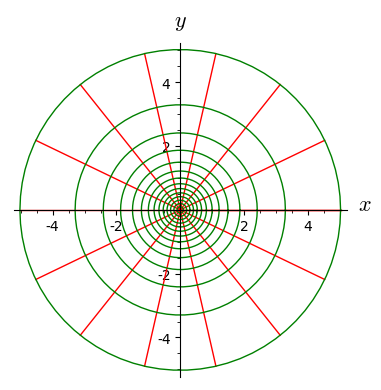Plot of stereographic coordinates in terms of spherical ones:

In :
stereoN.plot(chart=spher, number_values=15, plot_points=200,
color={x: 'blue', y: 'orange'})

Out: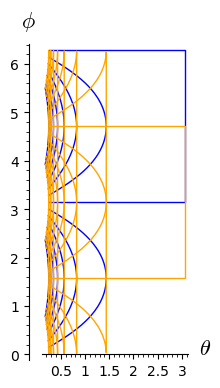The expression of the embedding $\Phi$ in terms of the stereographic coordinates is computed by Sage:

In :
Phi.display()

Out:

This means that we can use $\Phi$ for a 3D view of the stereographic chart:

In :
stereoN.plot(chart=cartesian, mapping=Phi, number_values=25,
color={x: 'blue', y: 'orange'})

Out:

The hole at the North pole is due to the default limitation to $[-8,8]$ for the plot range of coordinates that span the whole real line. We can change it to $[-20, 20]$ via the argument max_range:

In :
stereoN.plot(chart=cartesian, mapping=Phi, number_values=25,
color={x: 'blue', y: 'orange'},
max_range=20, plot_points=200)

Out: